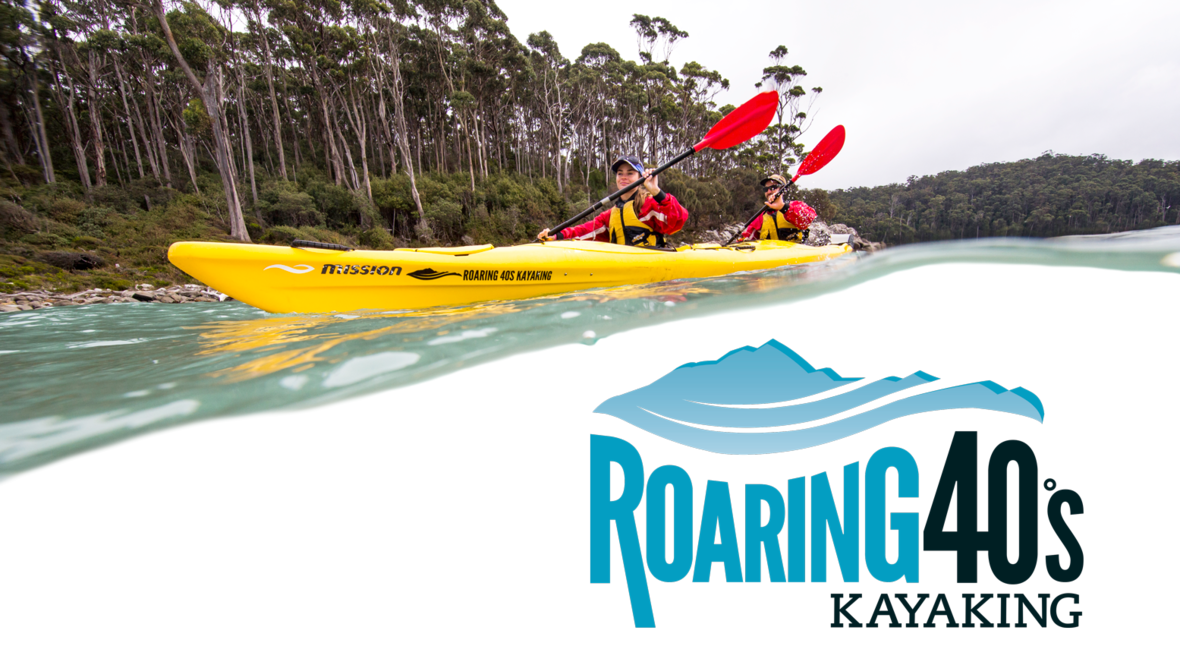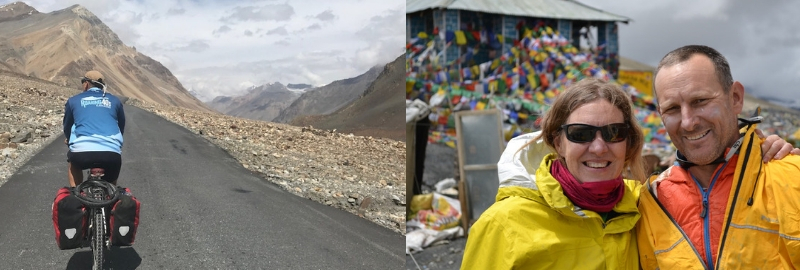Like   Tweettable div table+table+table+table div table{width:100%;padding:0}table div table+table+table+table div table img{width:96.23%;padding:0;float:none}table div table+table+table+table div table td{width:100%;padding:0 1.88% 18px}/* styles */## Expedition Kayaking

 table div table+table+table+table+table+table div table td,table.module-5{width:100%;padding:0}table div table+table+table+table+table+table div table{width:100%;float:none;margin-left:auto;margin-right:auto;padding:0}table div table+table+table+table+table+table div table a{border:0 none;text-decoration:none}table div table+table+table+table+table+table div table img{width:100%!important;border:0 none;text-decoration:none}/* styles */

## SOUTHWEST TASMANIAN EXPEDITIONS

If you’re dreaming of an adventure in a stunning, wild and remote place, Southwest Tasmania will fulfill that desire and then some. We love this wilderness and sharing the experience of being immersed in an untouched landscape with its challenges and breathtaking beauty. Paddlers on our expeditions talk of it a being life-changing experience, here are just a few of their comments:

“..all of this in a superlative natural area…Bliss. May it stay awesome always.”
“You will be grinning from ear to ear. After a week on dry land, we still are!”
“If you want to experience the real southwest wilderness of Tasmania, this is the trip for you.”

 table div table+table+table+table+table+table+table+table+table div table td,table.module-8{width:100%;padding:0}table div table+table+table+table+table+table+table+table+table div table{width:100%;float:none;margin-left:auto;margin-right:auto;padding:0}table div table+table+table+table+table+table+table+table+table div table a{border:0 none;text-decoration:none}table div table+table+table+table+table+table+table+table+table div table img{width:100%!important;border:0 none;text-decoration:none}/* styles */

## FLINDERS ISLAND EXPEDITIONS

The land and seascapes of Flinders Island are equally magnificent. The spectacular granite peaks that rise up out of the sea remain in sight as you skim across the blue waters. The wildlife on land and sea is abundant, the produce as fresh as you can get and, when you add in the friendly, laidback locals, it’s an island exploration you’re unlikely to forget. Last year's kayakers said:

"One of the best times ever and I'd go back again tomorrow!"
"Great trip, great guides, fabulous scenery and the weather added atmosphere!"
"What a fantastic trip ... it certainly had the wow factor."

 /* styles */ The first five expedition bookings (Southwest Tasmania or Flinders Island) to use the code SUPERLATIVE in the special instructions will receive a special pack valued at \$150 including a Roaring 40s Kayaking shirt. (Valid for bookings made between 13th September and 31st October 2018 ONLY)
 table div table+table+table+table+table+table+table+table+table+table+table+table+table+table div table{width:100%;padding:0}table div table+table+table+table+table+table+table+table+table+table+table+table+table+table div table img{width:96.23%;padding:0;float:none}table div table+table+table+table+table+table+table+table+table+table+table+table+table+table div table td{width:100%;padding:0 1.88% 18px}/* styles */## Reg's Pre-season Kayak Checklist

 table div table+table+table+table+table+table+table+table+table+table+table+table+table+table+table+table div table td,table.module-15{width:100%;padding:0}table div table+table+table+table+table+table+table+table+table+table+table+table+table+table+table+table div table{width:100%;float:none;margin-left:auto;margin-right:auto;padding:0}table div table+table+table+table+table+table+table+table+table+table+table+table+table+table+table+table div table a{border:0 none;text-decoration:none}table div table+table+table+table+table+table+table+table+table+table+table+table+table+table+table+table div table img{width:100%!important;border:0 none;text-decoration:none}/* styles */
 /* styles */ Reg, Tory and Tom are busy checking our gear for a summer of paddling (and they didn't even let a bit of snow slow them down!). Now is also the perfect time to check your own kayaks and equipment to make sure you’ll be ready the moment the summer paddling weather arrives. Reg has put together a checklist to help you out!
 table div table+table+table+table+table+table+table+table+table+table+table+table+table+table+table+table+table+table+table div table{width:100%;padding:0}table div table+table+table+table+table+table+table+table+table+table+table+table+table+table+table+table+table+table+table div table img{width:96.23%;padding:0;float:none}table div table+table+table+table+table+table+table+table+table+table+table+table+table+table+table+table+table+table+table div table td{width:100%;padding:0 1.88% 18px}/* styles */table div table+table+table+table+table+table+table+table+table+table+table+table+table+table+table+table+table+table+table+table+table div table td,table.module-20{width:100%;padding:0}table div table+table+table+table+table+table+table+table+table+table+table+table+table+table+table+table+table+table+table+table+table div table{width:100%;float:none;margin-left:auto;margin-right:auto;padding:0}table div table+table+table+table+table+table+table+table+table+table+table+table+table+table+table+table+table+table+table+table+table div table a{border:0 none;text-decoration:none}table div table+table+table+table+table+table+table+table+table+table+table+table+table+table+table+table+table+table+table+table+table div table img{width:100%!important;border:0 none;text-decoration:none}/* styles */
 /* styles */ From weekend skill sessions to 2 hour pool sessions, our upcoming instructional program has something for everyone. These sessions aim to give you the confidence and inspiration to get out and enjoy the water this summer. Kayaking skills weekend – two fun days of kayaking and camping covering advanced strokes, group management, route and trip planning, weather conditions and much more. Eskimo Roll – over 2 nights in a warm pool, learn to master the Eskimo roll. Rescue Pool Session – essential skills to manage a capsize situation. Intro to Sea Kayaking and safety aspects for sea kayaking. Kayak Sea Skills – full day of intermediate kayaking skills training.
 table div table+table+table+table+table+table+table+table+table+table+table+table+table+table+table+table+table+table+table+table+table+table+table+table div table{width:100%;padding:0}table div table+table+table+table+table+table+table+table+table+table+table+table+table+table+table+table+table+table+table+table+table+table+table+table div table img{width:96.23%;padding:0;float:none}table div table+table+table+table+table+table+table+table+table+table+table+table+table+table+table+table+table+table+table+table+table+table+table+table div table td{width:100%;padding:0 1.88% 18px}/* styles */## Coming up in Tassie/* styles */ So you’ve ticked the box for a kayaking adventure with us, what else can you do while you’re here? You can mountain bike, drink wine, buy up at the markets (see our blog posts for more on these) or visit these particularly Tasmanian events: The Unconformity, Queenstown 19-21 October - Contemporary arts festival on Tasmania’s Wild West Coast. A community with an unmatched sense of place. The National Picture, Tasmanian Museum & Art Gallery, Hobart - The art of Tasmania’s Black War. Freycinet Challenge, East Coast 6-7 October - Multisport event in spectacular location (with our very own Melzi competing too!) Sheffield Medieval Festival13-14 October - Jousting, sword combat and feasting! Bruny Island Bird Festival19-22 October - On the island home of all 12 endemic bird species. Bicheno Food & Wine Festival16-18 November - East Coast food, wine, sun and beaches!
 table div table+table+table+table+table+table+table+table+table+table+table+table+table+table+table+table+table+table+table+table+table+table+table+table+table+table+table+table+table div table{width:100%;padding:0}table div table+table+table+table+table+table+table+table+table+table+table+table+table+table+table+table+table+table+table+table+table+table+table+table+table+table+table+table+table div table img{width:96.23%;padding:0;float:none}table div table+table+table+table+table+table+table+table+table+table+table+table+table+table+table+table+table+table+table+table+table+table+table+table+table+table+table+table+table div table td{width:100%;padding:0 1.88% 18px}/* styles */## Five questions on cycling Manali to Lehtable div table+table+table+table+table+table+table+table+table+table+table+table+table+table+table+table+table+table+table+table+table+table+table+table+table+table+table+table+table+table+table+table+table+table div table{width:100%;padding:0}table div table+table+table+table+table+table+table+table+table+table+table+table+table+table+table+table+table+table+table+table+table+table+table+table+table+table+table+table+table+table+table+table+table+table div table img{width:96.23%;padding:0;float:none}table div table+table+table+table+table+table+table+table+table+table+table+table+table+table+table+table+table+table+table+table+table+table+table+table+table+table+table+table+table+table+table+table+table+table div table td{width:100%;padding:0 1.88% 18px}/* styles */table div table+table+table+table+table+table+table+table+table+table+table+table+table+table+table+table+table+table+table+table+table+table+table+table+table+table+table+table+table+table+table+table+table+table+table+table+table div table{width:100%;padding:0}table div table+table+table+table+table+table+table+table+table+table+table+table+table+table+table+table+table+table+table+table+table+table+table+table+table+table+table+table+table+table+table+table+table+table+table+table+table div table img{width:96.23%;padding:0;float:none}table div table+table+table+table+table+table+table+table+table+table+table+table+table+table+table+table+table+table+table+table+table+table+table+table+table+table+table+table+table+table+table+table+table+table+table+table+table div table td{width:100%;padding:0 1.88% 18px}/* styles */